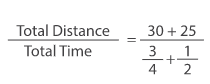# Sample Aptitude Questions of Mu Sigma

1. The average age of a man and his son is 28 years. The ratio of their ages is 3 :1 respectively. What is the man's age?
1. 30 years
2. 38 years
3. 40 years
4. 42 years
5. None of these

Total sum of man's age & his son's age =28 Ã— 2 = 56 Now, the Ratio of their ages is 3 : 1.Therefore, Man's age = (3/4) Ã— 56 = 42 So, the correct answer is option D.
So, the correct answer is option D.
2. A basket contains 3 blue, 5 black and 3 red balls. If 3 balls are drawn at random what is the probability that all are black?
1. 2/11
2. 1/11
3. 3/11
4. 8/33
5. None of these

Ways of selecting 3 black balls out of 5 - 5C3 Total ways of selecting 3 balls - 11C3 The required probability = (5C3 / 11C3 ) = (10/165) = (2/33)
1. The ratio of the capacity to do work of A and B is 3 : 2. If they together can complete a work in 18 days, then how long does A take to complete the work alone?
1. 45
2. 30
3. 24
4. 40
5. None of these

Let A and B take 3x and 2x days to complete the work
1/3x+1/2x=1/18 ;x=15
So A will take 45 days.
2. How many integers are there between 300 and 600 that are divisible by 9?
1. 33
2. 31
3. 28
4. 25
5. None of these

The sequence is 306,â€¦ 594 594=306+(n-1)9â‡’288=(n-1)9â‡’n=33
3. The sum of two numbers is 14. If the sum of their reciprocals is 7/24, find the numbers:
1. 4,8
2. 8,12
3. 4,10
4. 6,8
5. None of these

Going by option , option number 3 and 4 will give sum = 14 but 4th option will give l.c.m 24 and third option gives LCM as 20. It has to be the fourth option.
4. A, B, C and D are four consecutive odd numbers and their average is 42. What is the product of B and D?
1. 1860
2. 1890
3. 1845
4. 1677
5. None of these

As there are As diff. Is same so average should lie between B and C so B is 41 & C is 43 so D must be 45 as we have to find the product of B and D so it would be 1845.
5. The product of two alternative multiples of 4 is eight more than five times their sum. What are the two numbers?
1. 16,32
2. 12,20
3. 8,16
4. 16,24
5. None of these

Though options, 3rd option satisfies all the conditions.
6. Find the three terms in arithmetic series such that their sum is 30 and product is 910
1. 8,10,12
2. 7,10,13
3. 6,10,14
4. 5,13,14
5. None of these

Option 2nd gives product 910.
7. A car covers the first 30 km of its journey in 45 minutes and the remaining 25 km in 30 minutes. What is the average speed of the car?
1. 40km/hr
2. 64km/hr
3. 49km/hr
4. 48km/hr
5. None of these

Average speed == 44 km/hr So option E is the answer.